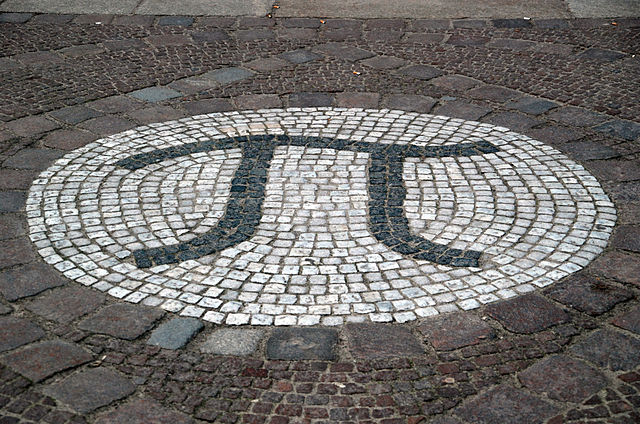Having just finished work on a major project milestone, I took it easy for a few days, allowing myself to spend time thinking about other things. That’s when I encountered an absolutely neat problem on Quora.Someone asked a seemingly innocuous number theory question: are there two positive integers such that one is exactly the π-th power of the other?

Now wait a minute, you ask… We know that π is a transcendental number. How can an integer raised to a transcendental power be another integer?

But then you think about $$\alpha=\log_2 3$$ and realize that although $$\alpha$$ is a transcendental number, $$2^\alpha=3$$. So why can’t we have $$n^\pi=m$$, then?

As it turns out, we (probably) cannot, but the reason is subtle and it relies on a very important, but unproven conjecture from transcendental number theory.

But first, let us rewrite the equation by taking its logarithm:

$$\pi\log n = \log m.$$

We can also divide both sides by $$\log n$$, which leads to

$$\pi = \frac{\log m}{\log n}=\log_n m,$$

but it turns out to be not very helpful. However, squaring the equation will help, as we shall shortly see:

$$\pi^2\log^2 n=\log^2 m.$$

Can this equation ever by true for positive integers $$n$$ and $$m$$, other than the trivial solution $$n=m=1$$, that is?

To see why it cannot be the case, let us consider the following triplet of numbers:

$$(i\pi,\log n,\log m),$$

and their exponents,

$$(e^{i\pi}=-1, e^{\log n}=n, e^{\log m}=m).$$

The three numbers $$(i\pi,\log n,\log m)$$ are linearly independent over $${\mathbb Q}$$ (that is, the rational numbers). What this means is that there are no rational numbers $$A, B, C, D$$ such that $$Ai\pi+B\log n+C\log m + D=0$$. This is easy to see as the ratio of $$\log n$$ and $$\log m$$ is supposed to be transcendental but both numbers are real, whereas $$i\pi$$ is imaginary.

On the other hand, their exponents are all rational numbers ($$-1, n, m$$). And this is where the unproven conjecture, Schanuel’s conjecture, comes into the picture. Schanuel’s conjecture says that given $$n$$ complex numbers $$(\alpha_1,\alpha_2,…,\alpha_n)$$ that are linearly independent over the rationals, out of the $$2n$$ numbers $$(\alpha_1,…,\alpha_n,e^{\alpha_1},…,e^{\alpha_n})$$, at least $$n$$ will be transcendental numbers that are algebraically independent over $${\mathbb Q}$$. That is, there is no algebraic expression involving roots and powers of the $$\alpha_i$$, $$e^{\alpha_i}$$, and rational numbers that will yield 0.

The equation $$\pi^2\log^2 n=\log^2 m$$, which we can rewrite as

$$(i\pi)^2\log^2 n + \log^2 m=0,$$

is just such an equation, and it can never be true.

I wish I could say that I came up with this solution but I didn’t. I was this close: I was trying to apply Schanuel’s conjecture, and I was of course using the fact that $$\pi=-i\log -1$$. But I did not fully appreciate the implications and meaning of Schanuel’s conjecture, so I was applying it improperly. Fortunately, another Quora user saved the day.

Still I haven’t had this much fun with pure math (and I haven’t learned this much pure math all at once) in years.